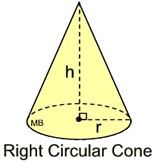## How to Calculate and Solve the Centre of Gravity of a Right Circular ConeThis image above is a display of what a right circular cone looks like. There is only one essential parameter for calculating the centroid or centre of gravity of a right circular cone. This parameter is the height of the cone (h).

The formula for calculating the the centroid or centre of gravity of a right circular cone is:

C.G. = h / 4

As always let’s solve an example.

Find the centroid or centre of gravity of a right circular cone where the height of the cone is 12cm.
This implies that:

h = height of the cone = 12

C.G. = 12 / 4
C.G. = 3

Therefore, the centroid or centre of gravity of the right circular cone is 3.

Nickzom Calculator – The Calculator Encyclopedia is capable of calculating the centre of gravity of a right circular cone at a distance from its base measured along the vertical axis.

To get the answer and workings to center of gravity or centroid of a right circular cone using the Nickzom Calculator – The Calculator Encyclopedia. First, you need to obtain the app.

You can get this app via any of these means: